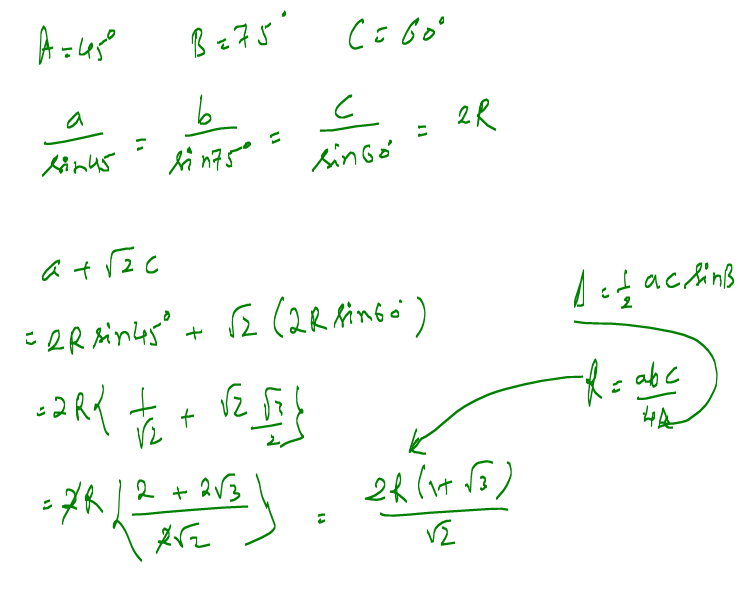Click to Chat

1800-1023-196

+91-120-4616500

CART 0

• 0

MY CART (5)

Use Coupon: CART20 and get 20% off on all online Study Material

ITEM
DETAILS
MRP
DISCOUNT
FINAL PRICE
Total Price: Rs.

There are no items in this cart.
Continue Shopping
`        if A=45,B=75, then a+c√2 is equal to,a)2bb)3bc)√2 bd)b `
3 years agoNishant Vora
IIT Patna
2467 Points
``````
3 years ago
```							a/sinA = b/sinB = c/sinC = ka= k/root2b= root3k/2a+ root2 b = k(1+root3)/root2 -----(eq 1) b/sinB =kb= sin 75 * kk= 2root b/(root3+1) Substitute value of k in equation 1) a + root c = 2b
```
2 years ago
```							a/sin45 = b/sin75 = c/sin60 = k a=k/√2.      b=ksin(45+30).         c=k√3/2                    b=k(√3+1)/2√2                i.e 2b=k(√3+1)/√2.  ------ (1)a+c√2 = k/√2 + k√3.√2/2            =k(1+√3)/√2            =2b.            (from 1)
```
25 days ago
Think You Can Provide A Better Answer ?

## Other Related Questions on Trigonometry

View all Questions »### Course Features

• 731 Video Lectures
• Revision Notes
• Previous Year Papers
• Mind Map
• Study Planner
• NCERT Solutions
• Discussion Forum
• Test paper with Video Solution### Course Features

• 31 Video Lectures
• Revision Notes
• Test paper with Video Solution
• Mind Map
• Study Planner
• NCERT Solutions
• Discussion Forum
• Previous Year Exam Questions